2018-10-15 16:05:11 hliyang 阅读数 105
• ###### 【Python】第16周 数据挖掘与机器学习进阶(上)

本周课程讲机器学习的概念，了解什么是机器学习以及机器学习的主要任务，kNN算法、线性回归算法的原理与实现，如何拆分训练数据集与测试数据集，算法中的超参数以及特征归一化等内容。实战部份，将讲解手写字体识别和房价预测。

613 人正在学习 去看看 CSDN就业班
###### k-近邻算法：

（kNN —— k-Nearest Neighbors）

###### 欧式距离公式：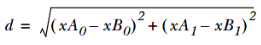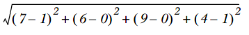###### 测试分类器的效果：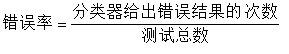###### 算法使用：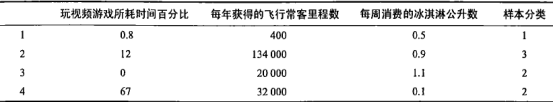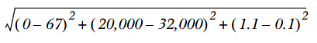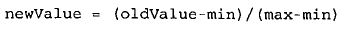min和max为数据集中对应特征的最小和最大值。

###### 算法特点：

k-近邻算法是基于实例的学习，使用算法时必须有接近实际数据的训练样本数据，同时又必须对数据集中的每个数据计算距离值，所以十分耗时。

###### 代码案例：

kNN算法应用实例（1、2）

knn 机器学习实战 相关内容

2019-04-15 10:58:14 starcosmos 阅读数 57
• ###### 【Python】第16周 数据挖掘与机器学习进阶(上)

本周课程讲机器学习的概念，了解什么是机器学习以及机器学习的主要任务，kNN算法、线性回归算法的原理与实现，如何拆分训练数据集与测试数据集，算法中的超参数以及特征归一化等内容。实战部份，将讲解手写字体识别和房价预测。

613 人正在学习 去看看 CSDN就业班

@[TOC]《机器学习实战》-KNN算法心得)

PS：第一次在CSDN上写，还不习惯这个编写方式

KNN是处理分类问题的，而且是监督的，即需要预先认为的给出一些已知类别的数据供KNN计算。其中对一个物体，需要人为的分成多个属性，并求出每个属性的值。它对属性的值要求可以是连续也可以是离散的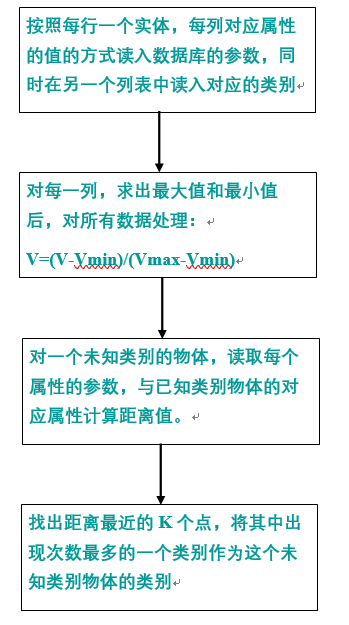KNN算法部分就到这里，接下来是决策树。

knn 机器学习实战 相关内容

2018-06-21 00:46:16 Evitachan 阅读数 750
• ###### 【Python】第16周 数据挖掘与机器学习进阶(上)

本周课程讲机器学习的概念，了解什么是机器学习以及机器学习的主要任务，kNN算法、线性回归算法的原理与实现，如何拆分训练数据集与测试数据集，算法中的超参数以及特征归一化等内容。实战部份，将讲解手写字体识别和房价预测。

613 人正在学习 去看看 CSDN就业班

KNN算法是监督学习分类方法。何为监督学习？我们用来训练的数据集应当包括数据特征和标签两个部分，通过训练建立数据特征和标签之间关系的算法模型，这样的话，将测试数据集套用算法模型，可以得到测试数据的标签。

在训练样本集中每个数据都存在标签，即我们知道样本集中每一数据与所属分类的对应关系。输人没有标签的新数据后，将新数据的每个特征与样本集中数据对应的特征进行比较，然后算法提取样本集中特征最相似数据（最近邻）的分类标签。选择k个最相似数据中出现次数最多的分类，作为新数据的分类。在KNN中，通过计算对象间距离作为各个对象之间的相似性指标，代替对象之间的匹配度计算。

对于训练样本数为m，特征数为n的训练样本集，计算测试样本xm个训练样本的欧氏距离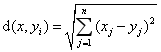其中i=12，……，m。对d(x,yi)进行降序排列，选择前k个值，出现次数最多的分类作为测试样本x的分类。

形象地理解，将训练样本和测试样本投影到n维空间上，以某个测试样本为中心，距离其最近的k个训练样本点采用投票原则，投出该训练样本的标签。

由此可见，k值的选择会影响到标签的决策。以iris数据集为例。```import numpy as np
import matplotlib.pyplot as plt
from sklearn import neighbors

# import some data to play with
from sklearn import datasets

# only take the first two features.
# we could avoid this ugly slicing by using a two-dim dataset
x = iris.data[:, :2]
y = iris.target

# k
n_neighbors = 3

# create color maps
from matplotlib.colors import ListedColormap
cmap_light = ListedColormap(['#FFAAAA', '#AAFFAA', '#AAAAFF'])
cmap_bold = ListedColormap(['#FF0000', '#00FF00', '#0000FF'])

# knn
n_neighbors = 3

for weights in ['uniform', 'distance']:
# we create an instance of Neighbors classifier and fit the data
clf = neighbors.KNeighborsClassifier(n_neighbors, weights = weights)
clf.fit(x, y)

# plot the decision boundary.
# For that, we will assign a color to each point in the mesh[x_min, x_max] * [y_min, y_max]
x_min, x_max = x[:, 0].min() - 1, x[:, 0].max() + 1
y_min, y_max = x[:, 1].min() - 1, x[:, 1].max() + 1
xx, yy = np.meshgrid(np.arange(x_min, x_max, .02), np.arange(y_min, y_max, .02))

# predict
z = clf.predict(np.c_[xx.ravel(), yy.ravel()])

# put the result into a color plot
z = z.reshape(xx.shape)
plt.figure()
plt.pcolormesh(xx, yy, z, cmap = cmap_light)

# plot also the training points
plt.scatter(x[:, 0], x[:, 1], c = y, cmap = cmap_bold, edgecolor = 'k', s = 20)
plt.xlim(xx.min(), xx.max())
plt.ylim(yy.min(), yy.max())
plt.title("3-Class classification (k = %i, weithts = '%s')" % (n_neighbors, weights))

plt.show()```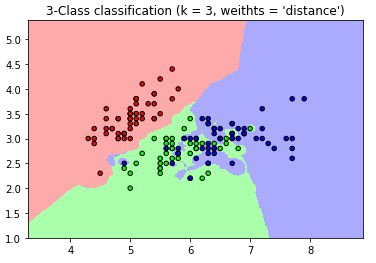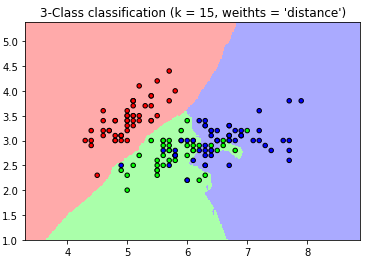比较两个图，可以很明显看出，当k取值不同时，分类的效果不同。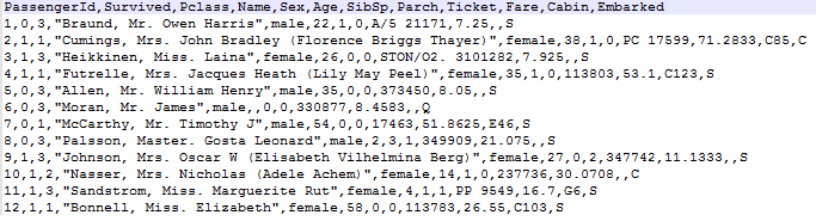```数据预处理
>训练集中共有891位乘客的数据信息，其中277位乘客的年龄数据缺失，余下数据年龄平均值为29.7，用30补全缺失项。
>性别男/女用1/0表示。```
```from numpy import *
import operator
from os import listdir

def classify0(inX, dataSet, labels, k):
dataSetSize = dataSet.shape
diffMat = tile(inX, (dataSetSize,1)) - dataSet
sqDiffMat = diffMat**2
sqDistances = sqDiffMat.sum(axis=1)
distances = sqDistances**0.5
sortedDistIndicies = distances.argsort()
classCount={}
for i in range(k):
voteIlabel = labels[sortedDistIndicies[i]]
classCount[voteIlabel] = classCount.get(voteIlabel,0) + 1
sortedClassCount = sorted(classCount.items(), key=operator.itemgetter(1), reverse=True)
return sortedClassCount

def createDataSet():
group = array([[1.0,1.1],[1.0,1.0],[0,0],[0,0.1]])
labels = ['A','A','B','B']
return group, labels

def file2matrix(filename):
file = open(filename)
numberoflines = len(arraylines) - 1
returnMat = zeros((numberoflines,3))    #创建行数为numberoflines,列数为2的矩阵
classLabel = []
index = 0
for line in arraylines[1:]:
line = line.strip()
lis = line.split(' ')
returnMat[index,:] = lis[0:3]
classLabel.append(int(lis[-1]))   #是为1类,否为0类
index += 1
return returnMat,classLabel

def autoNorm(dataSet):
minVals = dataSet.min(0)
maxVals = dataSet.max(0)
ranges = maxVals - minVals
normDataSet = zeros(shape(dataSet))
m = dataSet.shape
normDataSet = dataSet - tile(minVals, (m,1))
normDataSet = normDataSet/tile(ranges, (m,1))   #element wise divide
return normDataSet, ranges, minVals

def datingClassTest():
hoRatio = 0.1      #hold out 10%
datingDataMat,datingLabels = file2matrix('datingTestSet2.txt')
normMat, ranges, minVals = autoNorm(datingDataMat)
m = normMat.shape
numTestVecs = int(m*hoRatio)
errorCount = 0.0
for i in range(numTestVecs):
classifierResult = classify0(normMat[i,:],normMat[numTestVecs:m,:],\
datingLabels[numTestVecs:m],3)

str= "the classifier came back with: %d, the real answer is: %d"\
% (classifierResult, datingLabels[i])
print(str)
if (classifierResult != datingLabels[i]): errorCount += 1.0
str= "the total error rate is: %f" % (errorCount/float(numTestVecs))
print(str)```

使用交叉验证方法，选取一定比例数据作为测试集，比较利用KNN算法分类所得标签与真实标签结果是否一致，若不一致，则错误样本数加一。最终利用错误样本数除以总验证样本数得到错误率。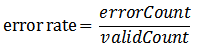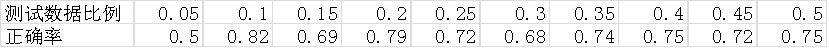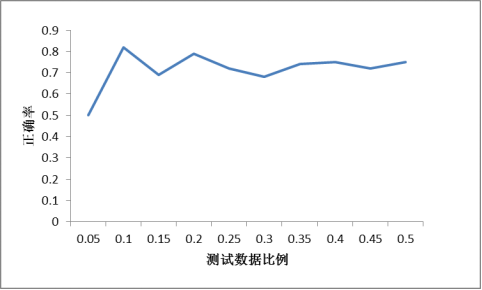1. 在选取10%数据作为测试数据时分类效果最好，这与最常用的十折交叉验证相符合。

2. k值选择过大或过小都会影响正确率，在使用KNN模型时，我们需要不断调整k值，直到找到局部最优解，这也是KNN算法需要解决的问题。

knn 机器学习实战 相关内容

2019-05-16 21:19:26 weixin_38286298 阅读数 4
• ###### 【Python】第16周 数据挖掘与机器学习进阶(上)

本周课程讲机器学习的概念，了解什么是机器学习以及机器学习的主要任务，kNN算法、线性回归算法的原理与实现，如何拆分训练数据集与测试数据集，算法中的超参数以及特征归一化等内容。实战部份，将讲解手写字体识别和房价预测。

613 人正在学习 去看看 CSDN就业班

# 机器学习实战之KNN实现

## KNN实现思想如下：

1）计算已知类别数据集中的点与当前点之间的距离；
2）按照距离递增依次排序；
3）选取与当前点距离最小的k个点；
4）确定前k个点所在类别的出现频率；
5）返回前k个点出现频率最高的类别作为当前点的预测分类；

## KNN优缺点

1. 精度高
2. 对异常值不敏感
3. 无数据输入假设（LR假设输入数据服从伯努利，线性回归假设输入数据服从高斯分布）

1. 计算复杂度高：需要和空间中每个点都计算距离（优化：kd树）
2. 空间复杂度高：需要存储所有点

## 代码实现

``````from numpy import *
import operator
#生成数据集
def createDataSet():
group = array([[1.0,1.1],[1.0,1.0],[0,0],[0,0.1]])
labels = ['A','A','B','B']
return group, labels
def knn(dataSet,labels,point,k):
#获得数据点个数
dataSetSize = dataSet.shape
#计算欧氏距离
#tile(key,(x,y))在行方向重复key x次，列方向重复key y次
diffMat = tile(point,(dataSetSize,1)) - dataSet
sqDiffMat = diffMat**2
sqDistances = sqDiffMat.sum(axis=1)
distances = sqDistances**0.5
#排序并返回原索引
sortedDistIndicies = distances.argsort()
class_count = {}
#计算统计前k次中，每个label次数
for i in range(k):
key = labels[sortedDistIndicies[i]]
#dic.get(key,0)获得key，若不存在则dic[key]=0
class_count[key] = class_count.get(key,0) + 1
sorted_class_count = sorted(class_count.iteritems(),key = lambda x:x,reverse=True)
return sorted_class_count

``````

knn 机器学习实战 相关内容

2018-10-11 10:00:10 fool_tds_rich 阅读数 31
• ###### 【Python】第16周 数据挖掘与机器学习进阶(上)

本周课程讲机器学习的概念，了解什么是机器学习以及机器学习的主要任务，kNN算法、线性回归算法的原理与实现，如何拆分训练数据集与测试数据集，算法中的超参数以及特征归一化等内容。实战部份，将讲解手写字体识别和房价预测。

613 人正在学习 去看看 CSDN就业班

# 机器学习 实战之KNN算法

## 一.KNN算法简介

KNN算法，即K - 近邻算算法，采用测量不同特征值之间的距离方法进行分类。它的工作原理是给算法输入一组训练样本集，该样本集每个数据都对应一个标签，而每条数据由若干个特征出现次数构成。待算法学习（读取）该组dataSet，用户便可输入一条新的数据，算法将新数据的每个特征与样本数据的每个特征进行比较，并且提取样本集中特征最相似的数据的分类标签，之后我们选择前K（K<=20）个最相似的数据Z中出现次数最多的标签作为该输入数据的标签。

## 二.算法核心-欧式距离计算

KNN算法使用计算输入数据与样本数据特征值间的欧式距离来判断两者的相似（近邻）程度，以此为基础来选择合适的前K个相似数据。其欧式距离公式如下：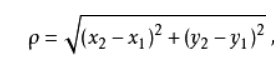## 三. KNN算法的执行

1. 计算已知欸别数据集中的点与当前输入数据点之前的距离
2. 按照距离递增的次序排列
3. 选取与当前点（待比较样本数据）距离最小的K个点
4. 确定前K个点所在类别的出现频率
5. 返回前K个点出现频率最高的类别作为当前嗲的预测分类

### 算法代码实现

``````from numpy import *
import operator
from os import listdir

def filematrix(filename):
fr = open(filename)
#获取数据的总行数n
numberofLines = len(arryLines)
returnMat = zeros((numberofLines,3)) #创建n行3列的数组，并且用0填充
classLabelVector = []
index = 0
#line每次循环会自增1
for line in arryLines:
#消除字符串头尾指定的字符（默认为空格或换行符）或字符序列，中间的不移除
#默认删除空白符(包括'\n','\r','\t',' ')
line = line.strip()
#通过指定分隔符对字符串进行切片，如果参数 num 有指定值，则仅分隔 num 个子字符串
#默认值为所有的空字符，包括空格、换行(\n)、制表符(\t)等
listFromLine = line.split('\t')
#注意，经过这两步操作，列表中（文件中）的数据前后的空距被消除,
#一行之间的多条数据也被拆分成单独的数据(还是按一行）存储在列表中
#如str = "Line1-abcdef \nLine2-abc \nLine4-abcd"，按‘\tab‘拆分后，
#为['Line1-abcdef', 'Line2-abc', 'Line4-abcd']

returnMat[index,:] = listFromLine[0:3]
classLabelVector.append(int(listFromLine[-1]))
index += 1
return returnMat,classLabelVector

def classify0(intx, dataSet, lables, k):
#dataSetSize 是用户输入需要分类的数据
#dataSet     是用户输入的样本数据
#sqDiffMat   是待分类数据与样本下每一个数据下X、Y的差值
#sqDistance  使用两点间距离公式计算距离

#numpy中shape返回数组的行数，shaepe返回数组的列数
#tile(inx,(dataSetSize,1))，将intx横向重复dataSetSize次，纵向重复1次
#例如inx=（【1，2】）--->（【1，2】，【1，2】，【1，2】）
dataSetSize = dataSet.shape
diffMat = tile(intx, (dataSetSize,1)) - dataSet
sqDiffMat = diffMat ** 2
sqDistance = sqDiffMat.sum(axis=1)
distances = sqDistance**0.5
#print ("distancs:",distances)
#返回distance中元素从小到大排序后的索引
sortDistance = distances.argsort()
#print("sortDistance:",sortDistance)
classCount = {}
for i in range(k):
#根据最短距离对应的样本元素索引取出前K个样本元素的类别
voteLable = lables[sortDistance[i]]
#print ("第%d个voteLable=%s:",i,voteLable)
#统计前K个元素类别出现的次数
#字典中的get（）方法，返回指定键对应的值，没有则返回默认值
classCount[voteLable] = classCount.get(voteLable,0)+1
#key=operator.itemgetter(0)根据字典的键进行排序，这也是默认排序
#key=operator.itemgetter(1)根据字典的值进行排序
#reverse降序排序字典
sortedClassCount = sorted(classCount.items(),key = operator.itemgetter(1),reverse = True)
#print ("sortedClassCount:",sortedClassCount)
return sortedClassCount

def autoNorm(dataSet):
#dataSet.min(0) 是从数据集中选取每列的最小值
minVals = dataSet.min(0)
maxVals = dataSet.max(0)
ranges = maxVals - minVals
#创建一个与dataSet列表相同尺寸的矩阵，以数字0填充
normDataSet = zeros(shape(dataSet))

#dataSet.shap 返回行数
m = dataSet.shape
normDataSet = dataSet - tile(minVals,(m,1))
normDataSet = normDataSet/tile(ranges,(m,1))
return normDataSet, ranges, minVals

def datingClassTest():
hoRatio = 0.10      #hold out 10%
datingDataMat,datingLabels = filematrix('datingTestSet2.txt')       #load data setfrom file
normMat, ranges, minVals = autoNorm(datingDataMat)
m = normMat.shape
numTestVecs = int(m*hoRatio)
errorCount = 0.0
for i in range(numTestVecs):
classifierResult = classify0(normMat[i,:],normMat[numTestVecs:m,:],datingLabels[numTestVecs:m],3)
print ("the classifier came back with: %d, the real answer is: %d" % (classifierResult, datingLabels[i]))
if (classifierResult != datingLabels[i]): errorCount += 1.0
print ("the total error rate is: %f" % (errorCount/float(numTestVecs)))

def imgvector(filename):
returnVect = zeros((1,1024))
fr = open(filename)
for i in range(32):
for j in range(32):
returnVect[0,32*i+j] = int(lineStr[j])
return returnVect

def handwritingClassTest():
hwLablels = []
#读取'trainingDigits'文件夹下的所有文件名，存储到trainingFileList列表中
trainingFileList = listdir('trainingDigits')
#获取‘trainingFileList’列表的长度m，即计算一共有多少个文件
m = len(trainingFileList)
#创建一个m行1024列的零矩阵，以便接下来每一行存储一个32*32的图像矩阵经i‘mgvector(filename)’函数处理过后的1*1024的向量
trainingMat = zeros((m,1024))
#for循环为了从文件名中解析出数字，即labels
for i in range(m):
#每一次循环读取‘trainingFileList【】’中的一个元素，即一个文件名‘XX_YY.txt’,并且存储到临时变量‘fileNmaeStr’中
fileNmaeStr = trainingFileList[i]
#使用numpy.split('.')函数将文件名‘XX_XX.txt’分割成 ‘XX-YY’, ‘txt’ 两部分，使用关键字【0】提取一个分量即‘xx_xx’存储到临时变量fileStr中
fileStr = fileNmaeStr.split('.')
#同理分离出'XX'存储到临时变量classNumStr中
classNumStr = int(fileStr.split('_'))
#将得到的文件类别存储下来
hwLablels.append(classNumStr)
#'%s'占位从C语言中借用，输出字符串，类似的还有'%d'，输出int类型。输出内容由 % 后面的内容决定,注意内容用（）括起来
trainingMat[i,:] = imgvector('trainingDigits/%s' % (fileNmaeStr))
testFileList = listdir('testDigits')
errorCount = 0.0
mTest = len(testFileList)
for i in range(mTest):
fileNmaeStr = testFileList[i]
fileStr = fileNmaeStr.split('.')
classNumStr = int(fileStr.split('_'))
vectorUnderTest = imgvector('testDigits/%s' % (fileNmaeStr))
classifierResult = classify0(vectorUnderTest, trainingMat, hwLablels, 3)
print("the classifier came back with: %d,the real answer is: %d" % (classifierResult,classNumStr))
if (classifierResult != classNumStr) : errorCount += 1.0
print ("\nthe total number of errors is: %d" % (errorCount))
print ("\nthe total number of error rate is: %f" % (errorCount/float(mTest)))

if __name__ == '__main__':
handwritingClassTest()

``````

knn 机器学习实战 相关内容